# How to Make Boxplots with Data Points using Seaborn in Python?

### Prerequisites :

Box Plot or a Whisker Plot is a statistical plot to visualize graphically, depicting group of numerical data through their quartiles. This plot displays the summary of set of data containing the five values known as minimum, quartile 1, quartile 2 or median, quartile 3 and maximum, where the box is drawn from first quartile to third quartile.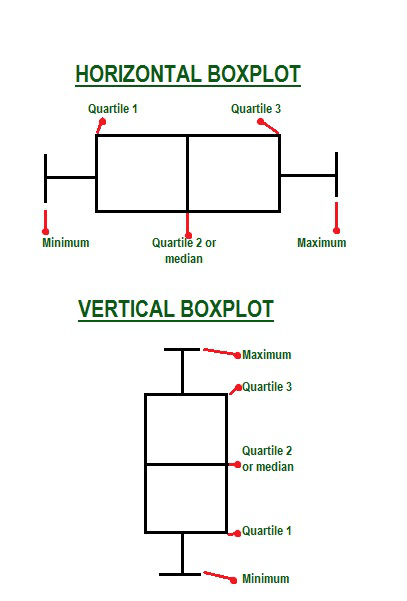A generic box plot mainly focuses on the five elements mentioned above to give the user a quartile based interpretation of the data, but it is possible to show data points on the box plot itself, making it more informative. For this seaborn is equipped with stripplot() function, all we have to do is call it just after boxplot() function with appropriate parameters to generate a boxplot with data points.

A strip plot is drawn on its own. It is a good complement to a boxplot or violinplot in cases where all observations are shown along with some representation of the underlying distribution. It is used to draw a scatter plot based on the category.

Syntax: seaborn.stripplot(*, x=None, y=None, hue=None, data=None, order=None, hue_order=None, jitter=True, dodge=False, orient=None, color=None, palette=None, size=5, edgecolor=’gray’, linewidth=0, ax=None, **kwargs)

Parameters:

• x, y, hue: Inputs for plotting long-form data.
• data: Dataset for plotting.
• order: It is the order to plot the categorical levels in.
• color: It is the color for all of the elements, or seed for a gradient palette

Returns: This method returns the Axes object with the plot drawn onto it.

### Approach:

• Import the library
• Create or load the dataset.
• Plot a boxplot using boxplot().
• Add data points using stripplot().
• Display plot.

Example 1: Regular box plot for comparison

## Python

 `# importing library ` `import` `seaborn as sns ` `import` `matplotlib.pyplot as plt ` ` `  `# loading seaborn dataset tips ` `tdata ``=` `sns.load_dataset(``'tips'``) ` ` `  `# creating boxplot ` `sns.boxplot(x``=``'size'``, y``=``'tip'``, data``=``tdata) ` ` `  `# display plot ` `plt.show() `

Output: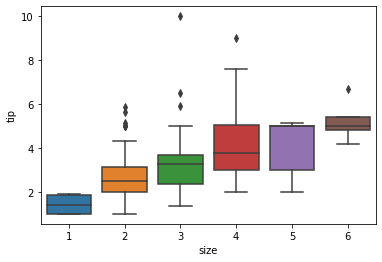Example 2: Creating box plot with data points

## Python

 `# importing library ` `import` `seaborn as sns ` `import` `matplotlib.pyplot as plt ` `# loading seaborn dataset tips ` `tdata ``=` `sns.load_dataset(``'tips'``) ` ` `  `# creating boxplot ` `sns.boxplot(x``=``'size'``, y``=``'tip'``, data``=``tdata) ` ` `  `# adding data points ` `sns.stripplot(x``=``'size'``, y``=``'tip'``, data``=``tdata) ` `# display plot ` `plt.show() `

Output: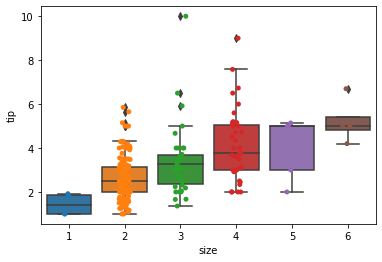Example 3: Boxplot with data points with non-default color

## Python

 `# importing library ` `import` `seaborn as sns ` `import` `matplotlib.pyplot as plt ` `# loading seaborn dataset tips ` `tdata ``=` `sns.load_dataset(``'tips'``) ` ` `  `# creating boxplot ` `sns.boxplot(x``=``'size'``, y``=``'tip'``, data``=``tdata) ` ` `  `# adding data points ` `sns.stripplot(x``=``'size'``, y``=``'tip'``, data``=``tdata, color``=``"grey"``) ` `# display plot ` `plt.show() `

Output: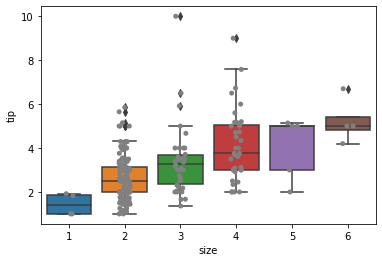Example 4: Changing size of the data points

## Python

 `# importing library ` `import` `seaborn as sns ` `import` `matplotlib.pyplot as plt ` `# loading seaborn dataset tips ` `tdata ``=` `sns.load_dataset(``'tips'``) ` ` `  `tdata ``=` `tdata.head(``10``) ` `# creating boxplot ` `sns.boxplot(x``=``'size'``, y``=``'tip'``, data``=``tdata) ` ` `  `# adding data points ` `sns.stripplot(x``=``'size'``, y``=``'tip'``, data``=``tdata, color``=``"grey"``, size``=``8``) ` `# display plot ` `plt.show() `

Output: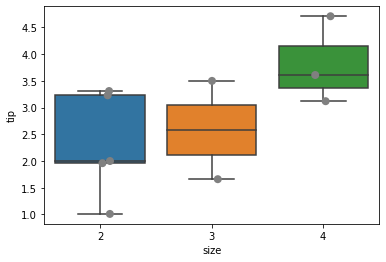Example 5: Plotting transparent data points

## Python

 `# importing library ` `import` `seaborn as sns ` `import` `matplotlib.pyplot as plt ` `# loading seaborn dataset tips ` `tdata ``=` `sns.load_dataset(``'tips'``) ` ` `  `tdata ``=` `tdata.head(``20``) ` `# creating boxplot ` `sns.boxplot(x``=``'size'``, y``=``'tip'``, data``=``tdata) ` ` `  `# adding data points ` `sns.stripplot(x``=``'size'``, y``=``'tip'``, data``=``tdata, color``=``"black"``, size``=``8``, alpha``=``0.5``) ` `# display plot ` `plt.show() `

Output: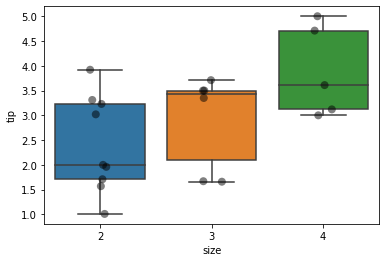Whether you're preparing for your first job interview or aiming to upskill in this ever-evolving tech landscape, GeeksforGeeks Courses are your key to success. We provide top-quality content at affordable prices, all geared towards accelerating your growth in a time-bound manner. Join the millions we've already empowered, and we're here to do the same for you. Don't miss out - check it out now!

Previous
Next